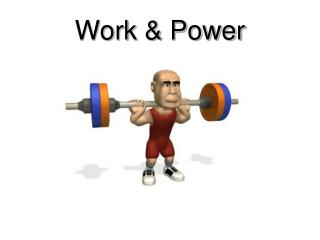DownloadDownload PresentationWork & Power

Work & Power

Télécharger la présentationWork & Power

- - - - - - - - - - - - - - - - - - - - - - - - - - - E N D - - - - - - - - - - - - - - - - - - - - - - - - - - -
Presentation Transcript

1. Work & Power

2. Force distance Work W = F d Work = Newton·Meters Work = Joules

3. Caution! Normal Force The force must be in the same direction as the distance moved or no work is done on the object. Since the force and distance moved are not in the same direction, no work is done on the tray! Distance Force (weight)

4. Effort vs Work

5. Have you done work on anything recently? Work I pushed a grocery cart How do you know you did work? How do you know you did work? Distance Force Distance Force I pushed horizontally The cart moved horizontally

6. 7 N Is work being done on the burrito? Yes! W = Fd W = (7 N)(1 m) W = 7 J 1 m

7. Is work being done on the egg? Normal Force Distance No work is done on the egg

8. Is work being done? (On the wall)

9. on the wall.

10. Work Power time Power is the rate at which work is done. P = W / t Power = Joules / seconds Power = Watts

11. How Powerful is she? When doing a chin-up, Courtney lifts her 425 N body a distance of 0.25 meters in 2 seconds. What is the power delivered by her biceps?

12. Work & Power Calculation Practice

13. What is the work done by Hajji who lifts a 30 kg bag straight up 1.5 meters? If it takes 0.5 seconds, what was his power rating? W = ? m = 30 kg d = 1.5 m t = 0.5 s P = ? W = Fd Fw = mg Fw = (30kg)(9.8 m/s2) Fw = 294 N W = (294 N)(1.5 m) W = 441 Nm or J P = W/t P = 441 J / 0.5 s P = 882 J/s or 882 Watts

14. How much time did it take for Marx to lift a 10 kg object 4 meters if his power rating was 160 Watts?

15. POWER LAB

16. Normal Force Weight Work The work done is: W = F d = force x distance = weight x height = (mg)(h)

17. 4th floor Where to? Group 7 Group 5 Group 6 3rd floor Group 4 Group 3 2nd floor Group 2 Group 1 1st floor

18. Where to? • Group 1: Stairs from 1st floor to middle 1st and 2nd • Group 2: Stairs from middle of 1st and 2nd to 2nd floor • Group 3: Stairs from 2nd floor to middle of 2nd & 3rd • Group 4: Stairs from middle of 2nd & 3rd to 3rd floor • Group 5: Stairs from 3rd floor to middle of 3rd and 4th • Group 6: Same as group 5 • Group 7: Stairs from middle of 3rd and 4th to 4th floor

19. Work, Power, Energy  Hockey • http://www.nbclearn.com/portal/site/learn/science-of-nhl-hockey

20. Simple machines can be used to change the direction of force Work = Force x Distance Work = 30 N x 4 m Work = 120 Nm or 120 J So the horse has done 120 J of work ON the bucket 30 N Bucket goes up a distance of 4 m Bucket’s weight 30 N

21. Images Cited • http://a.abcnews.com/images/WN/pd_scream_071012_ms.jpg • http://xpigs.files.wordpress.com/2007/12/latest-design-waiter-waitress-trays.gif • http://www.celticstrongman.co.uk/weightlifter_barbell_squats_hg_wht.gif